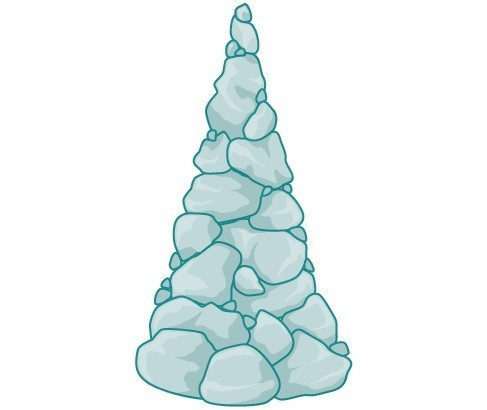## What you’ll learn to do: Evaluate the inverse of polynomial and radical functions

A mound of gravel is in the shape of a cone with the height equal to twice the radius.The volume is found using a formula from geometry.

\begin{align}V&=\frac{1}{3}\pi {r}^{2}h \\[1mm] &=\frac{1}{3}\pi {r}^{2}\left(2r\right) \\[1mm] &=\frac{2}{3}\pi {r}^{3} \end{align}

We have written the volume $V$ in terms of the radius $r$. However, in some cases, we may start out with the volume and want to find the radius. For example: A customer purchases 100 cubic feet of gravel to construct a cone shape mound with a height twice the radius. What are the radius and height of the new cone? To answer this question, we use the formula

$r=\sqrt{\dfrac{3V}{2\pi }}$

This function is the inverse of the formula for $V$ in terms of $r$.

In this section, we will explore the inverses of polynomial and rational functions and in particular the radical functions we encounter in the process.

## Contribute!

Did you have an idea for improving this content? We’d love your input.